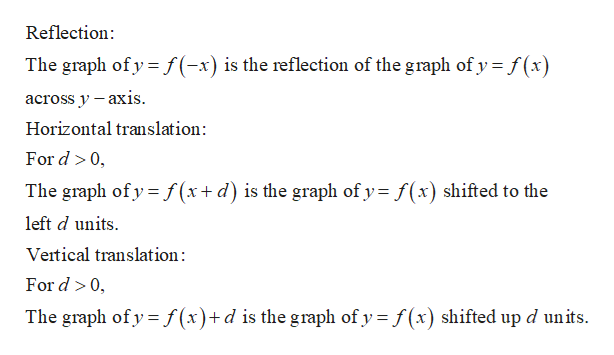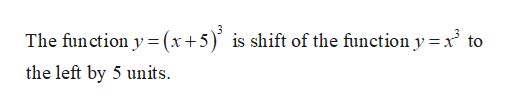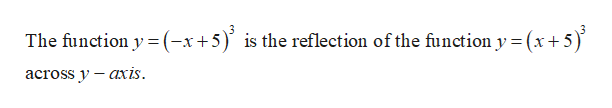# White a function based on the parent function y = x3 and transformation in given order:a) shift 5 units to the leftb) Reflect across the y-axisc) Shift upward 4 units

Question
1 views

White a function based on the parent function y = xand transformation in given order:

a) shift 5 units to the left

b) Reflect across the y-axis

c) Shift upward 4 units

check_circle

Step 1

Given:

The parent function y = x3.help_outlineImage TranscriptioncloseReflection The graph of y f(-x) is the reflection of the graph of y f(x across y -axis Horizontal translation: For d 0 The graph of y f (x+ d) is the graph of y f(x) shifted to the left d units Veitical translation: For d 0, The graph of y f(x)+d is the graph of y f(x) shifted up d units fullscreen
Step 2

Now perform the following transformations in order as follows.

a)help_outlineImage Transcriptionclose3 The fun ction y (x+5) is shift of the function y xto the left by 5 units fullscreen
Step 3

b)

...help_outlineImage Transcriptionclose3 The function y (-x+5)is the reflection of the function y (x5 х+ across y — ахis. fullscreen

### Want to see the full answer?

See Solution

#### Want to see this answer and more?

Solutions are written by subject experts who are available 24/7. Questions are typically answered within 1 hour.*

See Solution
*Response times may vary by subject and question.
Tagged in

### Algebra